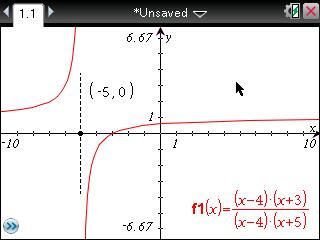# Knowledge Base

## Solution 29190: Creating a Vertical Line to Represent an Asymptote on theTI-Nspire™ Family Line of Products.

### How can I create a vertical line to represent asymptotes on the TI-Nspire family line of products?

The TI-Nspire family line of products does not have a built in way to represent asymptotes. However, if it is known that an asymptote exists please follow the steps below to draw a dashed line representing an asymptote.

Example: f1(X) = ((X-4) x (X+3)) / ((X-4) x (X+5)) has an asymptote at X=-5.

1) Press [home] [B]. Alternatively, click on "Add Graphs".2) Press [(] [(] [X] [-]  [)] [x].
3) Press [(] [X] [+]  [)] [)] [/].
4) Press [(] [(] [X] [-]  [)] [x].
5) Press [(] [X] [+]  [)] [)].
6) Press [enter].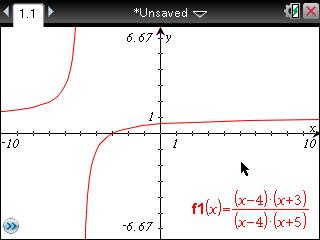7) Press [menu]   .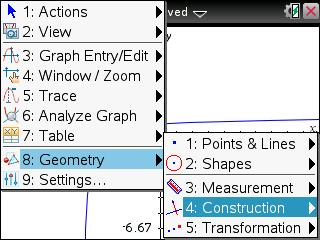8) Move the cursor to the approximate place the asymptote intersects the x-axis.
9) Press [enter] [enter].
10) A vertical line and a point on the x-axis will appear.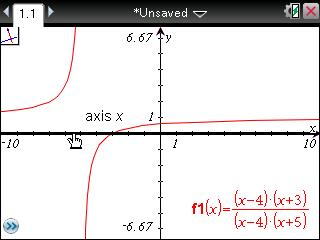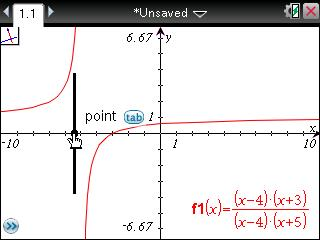11) To be more precise, press [menu]  .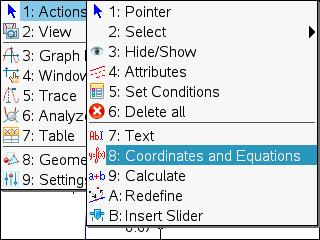12) Move the cursor over the point and press [enter].
13) Move the coordinates to a desired location and press [enter] [esc].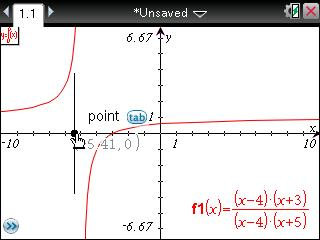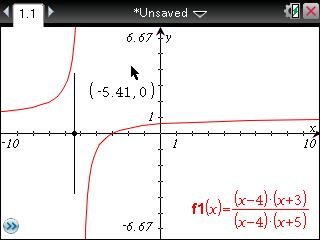14) Move the cursor to the x coordinate and press [enter] [enter].
15) Press [(-)]  [enter].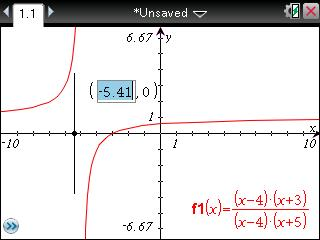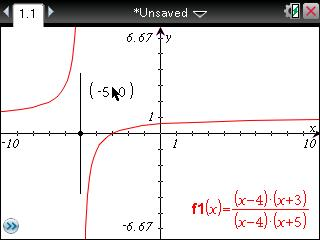17) Move to the vertical line and press [enter].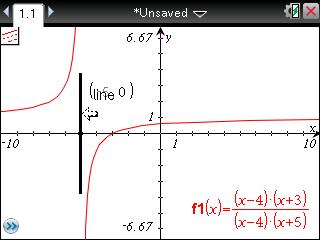18) Press [▼] [►] [►].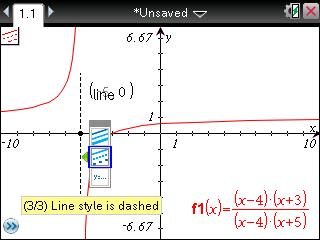19) Press [enter] [esc].